# Assignments

The assignments below are meant to practice the following:
a. calculating the equation of a line through a given point and with a given slope;
b. calculating the equation of a line through two given points;
c. calculating the tangent line at a point of a graph of a function.

1. Calculate the slope of the tangent line at the point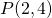of the graph of the function: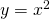Solution

2. Calculate the slope of the tangent line at the point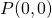of the graph of the function: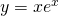Solution

3. Calculate the slope of the tangent line at the point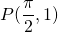of the graph of the function: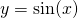Solution

4. Calculate the equation of the line through the point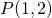with slope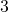.

Solution

5. Calculate the equation of the line through the point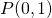parallel to the line: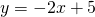Solution

6. Calculate the equation of the tangent line at the point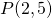of the graph of the function: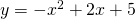Solution

7. Calculate the equation of the tangent line(s) of the graph of the function: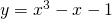parallel to the tangent line at the pointof the graph of the function:Solution

8. The line: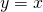intersects the parabola: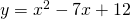in two points A en B.
The tangent lines at these points intersect in a point C.
Calculate the coordinates of C.

Solution

9. The graph of the function: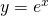intersects the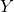-axis in point A.
The tangent line at this point of the graph intersects the-axis. Calculate the coordinates of this intersection point.

Solution

10. Give the equation of the line through the points:and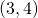Solution

0